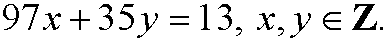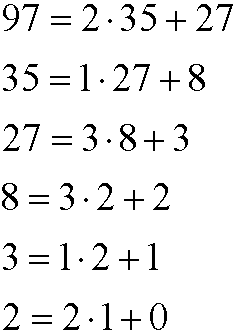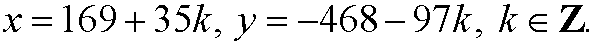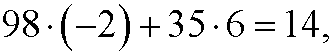# 4. Diophantine equations

diophantine equation is an equation where only integer solutions are accepted. This implies that diophantine equations becomes harder (or even impossible) to solve than equations that do not have this restriction. We will show that diophantine equations of the typea, b ,c, x, y integean be solved by Euclid’s algorithm (assuming that there is a solution). The method of solution depends of the coefficients a, b and c. We have three different variants that we treat in the examples below:
Example 1: Solve the equationFirst we examine gcd(97,35). We haveThis means that gcd(97,35)=1. Now we go backwards and express gcd(97,35) as a linear combination of 97 and 35:that isIf we multiply this equation by 13 we getwhich means that one solution of the diophantine equation is x=169, y=-468. This is however not the only solution. We can exactly as in part 3add and subtract the least common multiplesuch thatWe thus have infinitely many solutionsExample 2: Solve the equationFirst we examine gcd(98,35). We haveThis implies that gcd(98,35)=7, which means that every linear combination of 98 and 35 must contain the factor 7. Then also 98x+35ymust be a multiple of 7. But since the right hand side 13 does not contain the factor 7, there are no integer solutions to this equation.

Example 3: Solve the equationWe had from example 2 that gcd(98,35)=7. If we go backwards and express gcd(98,35) as a linear combination of 98 and 35 we get:that isIf we multiply this equation by 2 we getwhich implies that one solution to the diophantine equation is x=-2, y=-6. This is however not the only solution. We can exactly as in part 3 add and subtract the least common multiplesuch thatWe thus have infinitely many solutionswww.larserikpersson.se